Courses

Properties of Metals, Stress-Strain & Elastic Constants Notes | Study Strength of Materials (SOM) - SSC

SSC: Properties of Metals, Stress-Strain & Elastic Constants Notes | Study Strength of Materials (SOM) - SSC

The document Properties of Metals, Stress-Strain & Elastic Constants Notes | Study Strength of Materials (SOM) - SSC is a part of the SSC Course Strength of Materials (SOM).
All you need of SSC at this link: SSC

Properties of Metals

1. Ductility

• Ductility is the property by virtue of which material can be stretched to a reduced section under the action of tensile force.
• Large deformations are thus possible in ductile materials before the absolute failure or rupture takes place, some of the examples are mild steel, aluminium, copper, manganese, lead, nickel, brass, bronze, monal metal etc.

2. Brittleness

• Brittleness is the lack of ductility i.e. material can not be stretched. In brittle materials, failure takes place with a relatively smaller deformation. This property is undesirable. For brittle materials fracture point & ultimate points are same, and after proportional limit very small strain is seen. Some of the examples are  cast iron, concrete and glass.
• To distinguish between these two types of materials, materials with strain less  than 5% at fracture point are regarded as brittle and those having strains greater  than 5% at fracture point are called ductile. (This value for mild steel at fracture  is about 25%).

3. Malleability

• The property by which a material can be uniformly extended in all direction  without rupture. A malleable material possess a high degree of plasticity. This property is of great use in operations like forging, hot rolling, drop (stamping)  etc.

4. Toughness

• The property which enables materials to absorb energy without fracture. This  property is very desirable in case of cyclic loading or shock loading.
• The “Modulus of toughness” is measured as area under entire stress-strain  curve and is the energy absorbed by material of the specimen per unit volume  upto fracture stage.

Modulus of toughness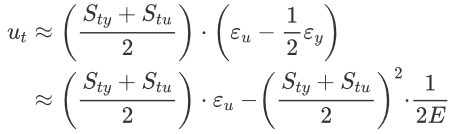where,

Sty is the tensile yield strength,

Stu is the tensile ultimate strength,

εy is the strain at yield,

εu is the ultimate strain (total strain at failure), and

E is the elastic modulus.

• The modules of toughness will depend upon ultimate tensile strength and strain at failure (fracture strain). Hence the material which is very ductile will exhibit a higher modulus of toughness as the case with mild steel.
• On the other hand the “Modulus of resilience” depends upon yield strength and hence a material with higher yield strength will have higher modulus of resilience
• The ‘Modulus of resilience’ is the maximum elastic energy per unit volume that  can be absorbed without attaining plastic stage.
Modulus of resilience (u)=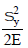•  Higher toughness a desirable property in materials used for gears, chains, crane  hooks, freight car etc. Higher resilience is desirable in springs.

5. Hardness

• Hardness is defined as the resistance to indentation or scratching or surface abrasion.
• Based upon this there are two methods of hardness measurement :
(i) Scratch hardness - Commonly measured by Mohr's test.
(ii) Indentation hardness (abrasion) measured by deformation.
1. Brinell hardness method
2. Rockwell hardness
3. Vickers hardness
4. Knoop hardness
• It should be noted that ductile materials are tough and brittle materials are hard.

6. Fatigue

• The behaviour of material under variable loads (dynamic loads) is referred to as “fatigue”. In recent past several failures of structures have been noted due to fatigue.
• Factors affecting fatigue are:
(iii) Corrosion
(iv) Temperature
(v) Stress concentration

7. Creep & Stress Relaxation

• At any temperature, a material will progressively deform with the passage of time under constant loading, even if the stress is below yield point, this phenomenon is called creep. However such deformation is negligibly small at lower temperature.
• At higher temperature, due to greater mobility of atoms, most of the material sloose their strength and elastic constants also get reduced. Hence greater deformation at elevated temperature results even under constant loading. Therefore creep is more pronounced at higher temperature, hence it must be considered for design of engines & furnaces.
• The temperature at which the creep becomes very appreciable is half of the  melting point temperature on absolute scale and is known as “Homologous temperature”.
• If a wire of metal is stretched between two immovable supports, so that it has  an initial tension stress so. The stress in the wire gradually diminishes, eventually  reaching a constant value. This process, which is a manifestation of creep, is  called “Stress relaxation”.

Stress-Strain Curve

Simple tension test for mild steel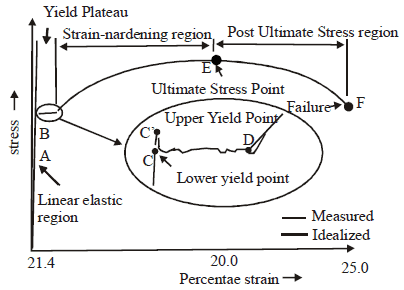• A is Limit of proportionality: Beyond A linear variation ceases.
• B is Elastic limit : The maximum stress up to which a specimen regains its original  length on removal of applied load. For mild steel B is very near to A. for other  materials B may be greater than A.
• C' is Upper yield point : The magnitude of the stress corresponding to C' depends  on the cross-sectional area, shape of the specimen and the type of the equipment  used to perform the test. It has no practical significance.
• C is lower yield point : The stress at C is the yield stress (σy) with a typical value  of σy = 250 N/mm2 for mild steel. The yielding begins at this stress.
• CD represents perfectly plastic region : It is the strain which occurs after the  yield point C, without any increase in stress. The strain corresponding to point D is  about 1.4% and corresponding to C is about 0.12% for mild steel. Hence plastic  strain is 10 to 15 times of elastic strain.
• DE represents strain hardening : In this range further addition of stress gives  additional strain, however strain increases with faster rate in this region. The material  in this range undergoes change in its atomic and crystalline structure, resulting in  increased resistance to further deformation. This portion is not used for structural  design.
• E is Ultimate point : The stress corresponding to this point is ultimate stress (σy)  and the corresponding strain is about 20% for mild steel.
• F is fracture point : Stress corresponding to this is called breaking stress & strain  is called fracture strain. It is about 25% for mild steel.
• Region between E & F is the necking region, in which area of cross-section is  drastically decreased.

Important Points to be noted

• Strain that occurs before the yield point is called elastic strain and that which occurs after yield point with no increase in stress is called plastic strain. For mild steel, Plastic Strain = 10 to 15 times of elastic strain.
• Ideal curve for tension is shown, however actual behaviour is different and indicates apparently reduced yield stress in compression for mild steel. The divergence between tension and compression results it is explained by Bauchinger and is called “Bauchinger effect”.
• The stress is defined as the ratio of load to original area (Ao) It is also known as ‘engineering stress’ or ‘stress’ or ‘nominal stress’.
Engg. stress ‘or’ Nominal stress =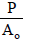• On the contrary when changing area is taken into account, the ratio of load to  actual area (A) is called ‘true stress’. True stress = (P/A)

Simple Stress and Strain

• It is the internal resistance offered by the body against external loading or  deformation. Stresses may be of following type :
(i) Direct stresses or normal stresses which may be tensile or compressive.
(ii) Shear or Tangential stresses.
(iii) Transverse or Bending stresses.
(iv) Torsional or Twisting stresses.
• For direct stresses, if area under consideration is original area, then it is known as
engineering stress or nominal stress or simply stress. But, if the area taken is actual
area, then stress is known as true stress.
Nominal Stress =True Stress = (P/A)
here,
Ao = Original area of specimen
A = Actual area of specimen
• When a prismatic bar is subjected to axial load, it undergoes a change in length. This change is length is usually called deformation. The deformation per unit length of the bar is termed as strain. Since strain is deformation per unit length, it is a dimensionless quantity. But sometimes in practice, strain is recorded in forms such as mm/m or μm/m etc.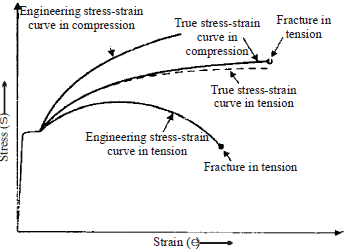Tension and Compression Monotonic Stress-strain Diagrams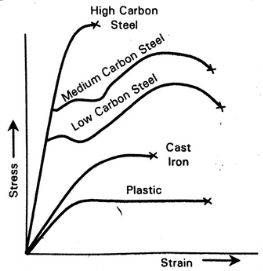Tensile stress-strain diagram for differents steels

1. Hooke’s Law

•  It states that within elastic limit stress for most of the metals is proportional to  strain.
Stress ∝ Strain
( Stress/Strain)= E(const.)
Where, E = Young’s modulus of elasticity.
• Hooke’s law is valid up to limit of proportionality. However for mild steel  proportional limit and Elastic limit are almost equal, but for other metals &  materials elastic limit may be higher than proportional limit, for example-rubber.
• The slope of stress-strain curve is called modulus of elasticity (E). The modulus of elasticity (E) is the constant of proportionality which is defined as the intensity of stress that causes unit strain. Thus, modulus of elasticity (E) has the units same as units of stress.

2. Proof Stress

• When a material such as aluminium does not have an obvious yield point and yet undergoes large strains after the proportional limit is exceeded, an arbitrary yield stress may be determined by the offset method.
• A line parallel to initial linear part is drawn, which is offset by some standard amount of strain such as 0.2%. The intersection of the offset point (A) defines the yield stress of offset yield stress, which is slightly above the proportional limit and is called proof stress.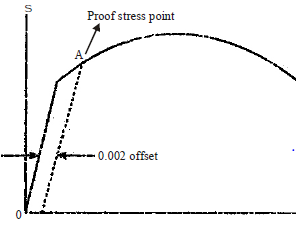Stress-strain curve for ‘aluminium’

Elongation of Bars
1. A bar of uniform cross-sectional area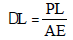2. Non-uniform bar
dL = (P/E)[L1/A1 + L2/A2+ _ _ _ _ _ ]
3. Tapering bar of circular cross-section whose diameter changes from ‘d1’ at one end to ‘d2’ at the other end.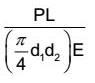where P is the load applied & E is the Young’s modulus of elasticity. L is the length of the specimen.
4. Tapering bar of rectangular cross-section having uniform thickness ‘t’ but width of the bar varies from ‘b1’ to 'b2'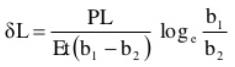5. Bars joined together parallel to each other

•  A structural member composed of two or more elements of different materials to form a parallel arrangement and subjected to axial loading is termed as a compound bar. Such a section is also known as composite section
•  Composite Young’s modulus is given by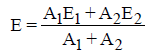Where A1 and E1 are properties of one bar and A2 and E2 are properties of other bar.
6. Elongation due to self weight
(a) For bar of uniform section (Prismatic bar): -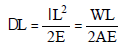Where λ = Unit - weight of the material
L= Length of the specimen
W = Total weight of specimen
A = Cross-sectional area.

•  Thus, the deformation of the bar under its own weight is half the deformation, if  the body is subjected to the direct load equal to the weight of the body.

(b) For bar of tapering section (Conical bar) :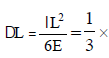Deflection of prismatic bar
OR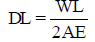7. Bar of Uniform Strength :

•  It is possible to maintain uniform stress at all the sections by increasing the area  from the lower level to higher level.
•  For a bar to have constant strength, the stress at any section, due to external  load and the weight of the portion below it should be constant i.e.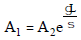Where Ais the area at upper section where the bar is fixed with immovable support. A2 is area at lower section which is freely hanging.

Temperature Stresses

• When a material undergoes change in temperature, its length also changes and if the  materials is free to expand or contract, no stresses are developed.
Free expansion in length is given by
ΔL = LαT
Where, L = Length of specimen
α = Coefficient of thermal change
T = Change in temperature
• If the free expansion or contraction is prevented then stresses will be developed,
which is given by
σ = αTE
• If the ends of the rod yield by an amount ‘d’, in this case the total amount of  expansion checked is given by
ΔL' = L α T – δ
• It may be noted that thermal stress or temperature stress does not depend upon the cross sectional area.

Temperature Stresses in Composite Sections

(a) When ends of a compound bar are free to expand

• If the temperature is increased then the metal with greater value of a will be in compression and the other metal will be in tension.
• On the other hand if the temperature is decreased then the nature of stresses will be change i.e., metal with greater value of a will be in tension and the other metal will be in compression.
Example: In copper and steel composite section, since coeff. of expansion of copper (αc) is greater than coefficient of expansion of steel (αs) hence free expansion of copper is more than that of steel.
• Let us take actual expansion of composite bar be ‘δ’ then L αc T > δ > L αs T.
Therefore steel is in tension and copper is in compression when the temperature is raised.
• Let the σs and σc are stresses in steel and copper. Then for equilibrium of system,
Total tension in steel = total compression in copper
σs As = σAc ...(i)
Also for composite section
Actual expansion of steel = Actual expansion of copper, this gives following condition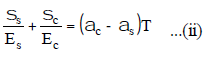Where Es and Ec are modulus of elasticity of steel and copper respectively.

Note: When ends of a compound bar are restrained, then on increasing the temperature metal with a greater value of a will be in compression and the other metal will be in tension and vice versa.

(b) When two bars are connected end to end between two fixed supports

• If the temperature is increased both the bars will undergo change in length. But due to the supports, the change in length is restrained.
• Hence both the bars will experience compressive stresses. If the temperature is decreased then both the bars will experience tensile stresses.
Total compressive force = σs As = σAc ...(i)
Total extension = 0
Therefore
σsLs/E+ σcLc/Es + ΔT(αsLs + αcLc) = 0

Elastic Constants

1. Poisson’s ratio (μ)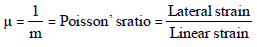The value of m lies between 3 and 4 for most of the metals.
μ = 0.05 to 0.1 for glass
= 0.1 to 0.2 for concrete
= 0.25 to 0.42 for metals
= 0.5 for pure rubber (for perfectly plastic body)

2. Volumetric strain (Îv)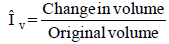•  For rectangular bar having, length = l, width = b, and thickness = d. The volumetric strain is given by.

or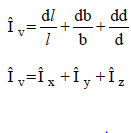For cylindrical rod, having diameter = d.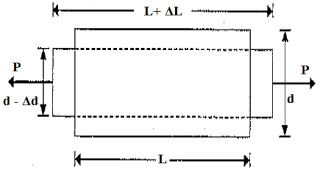Let,

L = Length of the cylindrical rod

d = Diameter of the cylindrical rod

Volume of the cylindrical rod, V = (Π/4) d2. L

ΔL= Change in length of the cylindrical rod

Δd= Change in diameter of the cylindrical rod

ΔV= Change in volume of the cylindrical rod

Let us determine the final dimensions of the cylindrical rod

Final length of the cylindrical rod = L+ ΔL

Final diameter of the cylindrical rod = d - Δd

Final volume of the cylindrical rod = (Π/4) (d - Δd) 2. (L+ ΔL)

Change in volume of the cylindrical rod = Final volume – initial volume

Change in volume of the cylindrical rod, ΔV = (Π/4) [(d2.L – 2.Δd.L.d + ΔL. d2)-(d2.L)]

ΔV= (Π/4) [ΔL. d2– 2.Δd.L.d]

Volumetric strain also known as bulk strain will be determined as following

Ԑv= Change in volume /original volume

Ԑv= dV/V

Ԑv= [(Π/4) (ΔL. d2– 2.Δd.L.d)]/ [(Π/4) d2.L]

Ԑv= [(ΔL. d2– 2.Δd.L.d)/ (d2.L]

Ԑv= (ΔL/L) – 2(Δd/d)

3. Shear Modulus

• When a body is subjected to shearing stresses, the shape of the body gets distorted. The measurement of this distortion is done by angle of distortion. Under pure shear the shape of the body gets distorted but the volume remains same.
• Modulus of rigidity.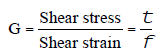Shear modulus is also known as modulus of rigidity.
• If under the shear, the shear strain is f then the linear strain in the diagonal of the  specimen is given by Î = (f/2)
i.e. linear strain of diagonal is half of the shear strain in the body.
• A shear stress in a given direction cannot exist without a balancing shear stress  of equal intensity in a direction at right angles to it. This stress is called  complimentary shear stress.

Relationship Between Elastic Constants

Young’s modulus,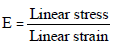Modulus of rigidity,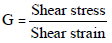Bulk modulus,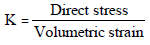Poisson’s ratio,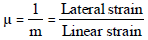Relationships: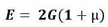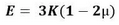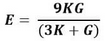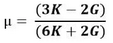Strain Energy

•  The strain energy is equal to the work done by the load provided no energy is added or subtracted in the form of heat. It is denoted by U. Thus,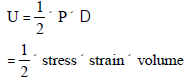For a prismatic bar,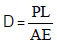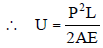•  The unit of strain Energy in SI units is Joule (J) which is equal to 1 N-m.
•  Resilience is also known as strain energy density. It represents the ability of the  material to absorb energy within elastic limit.  It is denoted by ‘u’. Thus,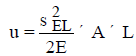•  When the stress (s) is equal to proof stress (sf) at the elastic limit, the corresponding resilience is known as proof resilience. Thus,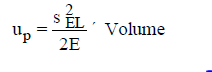•  Modulus of Resilience : - Proof Resilience / Volume =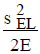•  Strain energy of a prismatic bar with varying section is given by
U = (p2/2E)[L1/A1 + L2/A2 + _ _ _ _ ]
•  Strain energy of a prismatic bar hanging under its own weight is given by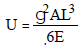where 'g' is the weight of bar per unit volume.
• Strain energy of a hanging elastic body due to more than one load cannot be found merely by adding the energies obtained from individual loads. This is because of the fact that strain in such a case is not a linear function but is a quadratic function of the loads.
The document Properties of Metals, Stress-Strain & Elastic Constants Notes | Study Strength of Materials (SOM) - SSC is a part of the SSC Course Strength of Materials (SOM).
All you need of SSC at this link: SSCUse Code STAYHOME200 and get INR 200 additional OFF Use Coupon Code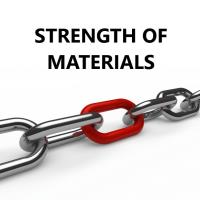Strength of Materials (SOM)

35 videos|33 docs|48 tests

Track your progress, build streaks, highlight & save important lessons and more!

,

,

,

,

,

,

,

,

,

,

,

,

,

,

,

,

,

,

,

,

,

,

,

,

;